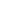There's An Arrow In The Logic- or -Maybe Pie Are Square, Maybe Notby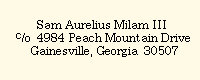This essay was first completed on Thursday, November 15, 1990 and was most recently revised on Thursday, June 7, 2018. This document is approximately 2,692 words long. Other essays in this collection are available on Pharos. This essay is LiteraShare. That means that it isn't for sale and that it isn't protected by a formal establishment copyright.  As the author, I ask you to extend to me the courtesy that is reasonably due.  If you copy this essay, then copy all of it, including my name and address as shown on each page, and this LiteraShare Statement.  I invite you to provide such copies for other readers.  If you quote from the essay, then do so accurately and give me credit.  If you care to make a voluntary contribution to me, then I prefer cash.  For checks, money orders, or PayPal payments, please inquire.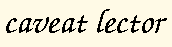There's An Arrow In The Logic - or - Maybe Pie Are Square, Maybe Not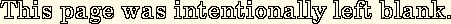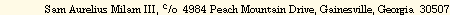There's An Arrow In The Logic - or - Maybe Pie Are Square, Maybe Not

The Quandary of the Arrow
 I shot an error into the air. It fell to earth most everywhere.  With thanks to Henry Wadsworth Longfellow
Have you heard the Zeno's dichotomy paradox?  According to that paradox, it's impossible to complete a journey or, when the paradox is stated differently, it's impossible to start a journey.  Zeno's arrow paradox is a different paradox but I like to apply the dichotomy paradox to arrows, in place of the arrow paradox.  The dichotomy paradox applies equally well to either situation.

So, according to Zeno's dichotomy paradox, as applied to the flight of an arrow, it's impossible for an arrow to reach its target.  The reasoning was that, whatever the distance between the bow and the target, the arrow has to first fly half of that distance, having then before it the remaining half.  It then again has to cover half of the remaining distance, having then before it half of the previous half.  The quandary arose from the facts that, according to Zeno's dichotomy, as applied to arrows, a flying arrow must always cover the first half of the distance to its target before it can cover the total distance and that the remaining distance, however small, can always be divided into half.  Thus, the arrow can't ever cover all of the distance.  It can't ever reach its target.

Any competent bowman can successfully argue the issue with a mathematician.  Presumably, that's what happened, and the mathematicians who were smart enough to concede the point (if you get my point) lived long enough to rethink the problem.  In fact, the mathematical description included some true facts but it didn't model the real world.  An arrow doesn't perform in terms of distance traveled per total distance.  It performs in terms of distance traveled per unit of time.  The flight of an arrow isn't based on proportions, but on speed.

There are some things to be learned from the Zeno's dichotomy paradox.  One of those things is that mathematics doesn't prove things.  Mathematics is descriptive.  It doesn't prove.  It describes.  It isn't possible to prove something mathematically.  Many untrue things have been "proved" mathematically and the cherished proofs have always been discarded when reality intervened.

Another thing to be learned from the dichotomy paradox is that a mathematical description must account not only for selected facts but also for the entire process, including all observed results.  That means that understanding must proceed not from the mathematical description of a process, but from the process.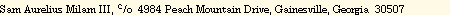Page 1

There's An Arrow In The Logic - or - Maybe Pie Are Square, Maybe NotPage 2There's An Arrow In The Logic - or - Maybe Pie Are Square, Maybe Not

Axiom Me No Questions, I'll Tell You Null Ayes

A commonly accepted (alleged) axiom of mathematics is that

1 / 0 =Ask most people whatmeans, and they'll tell you infinity. Wow.  How did we ever arrive at that?  Maybe something like this.

1 / 1 = 1

1 / .1 = 10

1 / .01 = 100

1 / .001 = 1000

1 / .0001 = 10,000

You get the idea.  You can make the denominator however small you want it to be and that will cause the result to be however large you want it to be.

The above series seems to suggest that

1 / 0 =just because the denominator in that series is asymptotic to zero.  However, the series doesn't say anything at all about division by zero.  No matter how small you make the denominator, it will never be zero.  How, then, can the series prove anything about division by zero?  This is a lot like the arrow not reaching the target.  Something's wrong with the relationship between the description and the real world.  If the denominator never arrives at zero, then the series doesn't describe division by zero.

As with the quandary of the arrow, an understanding of division by zero must proceed not from an inappropriate mathematical description but from the process of dividing things in the real world.  The conventional example is a pie.  Since I happen to like coconut cream pie, that's the kind that we'll use.

Suppose that I have a coconut cream pie and that I divide it into four pieces.  A mathematician would have you believe that

1 / 4 = .25.

However, let's see what each part of that equation really means.  In the real world, the equation might be "decoded" as

1 coconut cream pie / into four pieces = one fourth of a coconut cream pie.Page 3

There's An Arrow In The Logic - or - Maybe Pie Are Square, Maybe Not

The numerator tells you that I started with a whole quantity of something, in this case, a coconut cream pie.  The denominator tells you that I divided it into a certain number of portions, in this case, four of them.  The result is always misinterpreted.  It doesn't tell you that I have one fourth of a pie remaining, which is what people always believe it to mean.  It tells you two things.  One of them is obvious and the other one is not so obvious.  The obvious thing that it tells you is the size of one portion, after division.  In this case, a resulting portion is one fourth of a coconut cream pie.  This may seem absurdly simple for such a long explanation, but it isn't, because the result doesn't deal with all of what I have remaining.  There are still three other pieces of pie somewhere and therein lies the not so obvious part.  The result doesn't tell you how much pie there was, or is.  It tells you the size of one of the portions based on the number of portions defined in the denominator.  This means that the equation can model only instances wherein all portions are the same size.  Otherwise, the answer is ambiguous.  When the portions are of different sizes, then you don't know which one is described by the result.  Furthermore, when they are of different sizes, there is an unlimited number of possible results of the calculation.  Therefore, a better way to write the equation would be

1 / 4 = .25 + .25 + .25 + .25,

but that would confuse the mathematicians.  They confuse easily.

It's important to realize that the denominator can only describe a number of equal portions into which a whole is to be divided.  Furthermore, the whole quantity continues to exist after the division, even though it doesn't appear in the result, as shown using conventional notation.  And finally, it's important to remember that the result describes only the size of one portion and doesn't reveal the number of portions.  That was given in the denominator.

There's an important lesson in this.  The lesson is that, just as the rules of grammar and spelling can be used to write fiction, so can the rules of mathematics.  That is, one pie divided by .01 doesn't describe a thing that can possibly happen in the real world.  A fractional denominator doesn't describe a number of portions.  The concept of a fractional denominator is meaningless in the real world.  The result of such a calculation doesn't describe the size of a resulting portion because there can't be such a thing as a fractional portion in the real world.  Division by a fractional denominator is mathematically valid, but logically absurd.

 Page 4There's An Arrow In The Logic - or - Maybe Pie Are Square, Maybe Not

Profound Groundless Bounds Abound

1 / 0 =is the limit of the series shown earlier, and that the alleged limit is undefined, are wrong twice.  First, they're wrong because that isn't the limit of the series.  The series doesn't have a limit.  The denominator can get however small you want to make it.  That means that there's no limit to how small it can get.  Remember that small means a little bit of something.  Zero isn't a small quantity of something.  Zero is none of something.  Therefore, zero isn't a part of the series.  This means that small and zero are fundamentally different and can't be compared in the way that people try to compare them when they call one the limit of the other.  That the denominator appears to approach zero is meaningless in terms of relating division by a small number to division by zero.  No matter how small the number gets, it never becomes zero, so the two don't have anything to do with one another.

I can see those mathematicians now.  They're sitting there chortling, saying, "Oh, yeah?  Zero's got to be the limit 'cause tha series don't git no smaller then zero and negative numbers is smaller than zero!  Ha ha ha!"  Sorry guys.  We're talking about the real world.  In the real world, a negative number isn't smaller than zero.  In the real world, zero is the smallest possible number.  No matter how much you owe, for example, you can't have less money than none.  Your debt isn't a negative number, it just seems like it to you.  Your creditor considers your debt to be an asset, so "negative" is just the result of looking at something from the other direction.  Deceleration (negative acceleration) is positive acceleration in the other direction, and so forth.  Negative numbers are mathematically convenient and politically necessary, but fictional.  This shows not only mathematics, but politics and finance, in a hole nether light, doesn't it?

In fact, the entire series is undefined.  In the real world, things can be divided into numbers (of portions) which are larger than the number (of portions) with which you started.  That's what division means.  When you divide a thing, you get more portions.  Something can't be divided into fewer portions than you originally had.

An exception to this might seem to arise when you start with a numerator greater than one.  What if I have 12 chocolate chip cookies.  Then, for example,

12 / 2 = 6.Page 5

There's An Arrow In The Logic - or - Maybe Pie Are Square, Maybe Not
Doesn't that seem like I ended up with fewer portions than I originally had?  It might seem like it, but it isn't true.  Think about what really happened, and describe it using that unconventional notation that I suggested earlier.  You remember, the way that confuses the mathematicians.

Although the numerator is 12 and the result is 6, the number of portions in the result is still larger than the number of portions in the numerator.  In the result there are 2 portions, with 6 cookies per portion.  In the numerator there is one portion, with 12 cookies per portion.  Remember the meaning of the result.  The result isn't the number of portions.  It's the size of one portion.

The second way in which those folks are wrong (about eight or nine paragraphs back, I believe), is in declaring 1 / 0 to be an undefined calculation.  We're almost ready to define it.  First, however, let's be careful of the meaning of the symbol (=).  Remember the example,

1 / 4 = .25

where 1 means that I started with one pie, 4 means that I divided it into four equal pieces, and .25 means that each piece is one quarter of a pie.  Really, I still have an entire coconut cream pie.  That equal sign is very tricky.  Remember, what it really means is,

1 / 4 = .25 + .25 + .25 + .25.

If we want to use conventional notation, then we have to read the equal sign as meaning "... gives you ____ pieces of pie, each equal to ____ the size of the original pie", where the denominator goes in the first blank and the result goes in the second blank.  Then, we can do another series.
 One pie divided into four pieces gives you four pieces of pie, each equal to one quarter of the size of the original pie.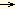1 / 4 = .25 One pie divided into three pieces gives you three pieces of pie, each equal to one third of the size of the original pie.1 / 3 = .3333 etc. One pie divided into two pieces gives you two pieces of pie, each equal to one half of the size of the original pie.1 / 2 = .5 One pie divided into one piece gives you one piece of pie, equal to the size of the original pie.1 / 1 = 1 One pie divided into 0 pieces ....?
Wait a minute.  In the real world, what does it mean to have zero pieces?  Zero doesn't mean a portion, not even a small portion.  It doesn't mean all of the pie or an infinite amount of pie.  It means that you don't have any pie.  Thus, if division by zero can

 Page 6There's An Arrow In The Logic - or - Maybe Pie Are Square, Maybe Not

have any meaning at all, then it must mean that the result is zero.  Therefore, the end of this series, when the denominator gets as small as it's possible for it to get, is
 one pie divided into 0 pieces means that there isn't any pie.1 / 0 = 0
If you're a mathematician, then by now you're probably screaming.  However, you can't prove me wrong because the only tool that you have is mathematics, which can't prove anything.  It only describes.  It doesn't prove.  You may continue to ponder this if you wish, or you may concede to the bowman.  I'm going to go divide a pie by zero.

Quod erat demonstrandum.Page 7

There's An Arrow In The Logic - or - Maybe Pie Are Square, Maybe NotPage 8There's An Arrow In The Logic - or - Maybe Pie Are Square, Maybe Not

AfterwardThose mathematicians who still believe that it's impossible to divide by zero shouldn't feel too bad about it.  They're following in a long tradition.  Here are a very few of the many illustrious others who've preceded them, and some of the things about which they were wrong.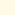Vannevar Bush (1890-1974)"There has been a great deal said about a 3,000 mile rocket.  In my opinion such a thing is impossible for many years.  I say technically I don't think anyone in the world knows how to do such a thing and I feel confident it will not be done for a very long period of time.  I think we can leave it out of our thinking."
 Testimony before the Senate, December, 1945Lee De Forest (1873-1961)Holder of more than 300 patents"While theoretically & technically television may be feasible, commercially and financially I consider it an impossibility, a development of which we need waste little time dreaming."
 (speaking in 1926)William Daniel Leahy (1875-1959)Admiral in the navy, Distinguished Service Medal, 1939"That is the biggest fool thing we have ever done ... the [atomic] bomb will never go off, and I speak as an expert in explosives."
 Speaking to President Truman in 1945, presumably prior to August 6Robert Andrews Millikan (1868-1953)Comstock Prize of the National Academy of Sciences, 1913
Nobel Prize in Physics, 1923"There is no likelihood man can ever tap the power of the atom."Ernest Rutherford (1871-1937)Nobel Prize for chemistry, 1908"The energy produced by the breaking down of the atom is a very poor kind of thing.  Anyone who expects a source of power from the retransformation of these atoms is talking moonshine."Cheer up.  Nobody's perfect.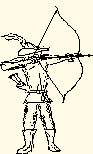Page 9

There's An Arrow In The Logic - or - Maybe Pie Are Square, Maybe NotPage 10Back to the Directory of Essays about Math and Science Back to the Beginning of This Document